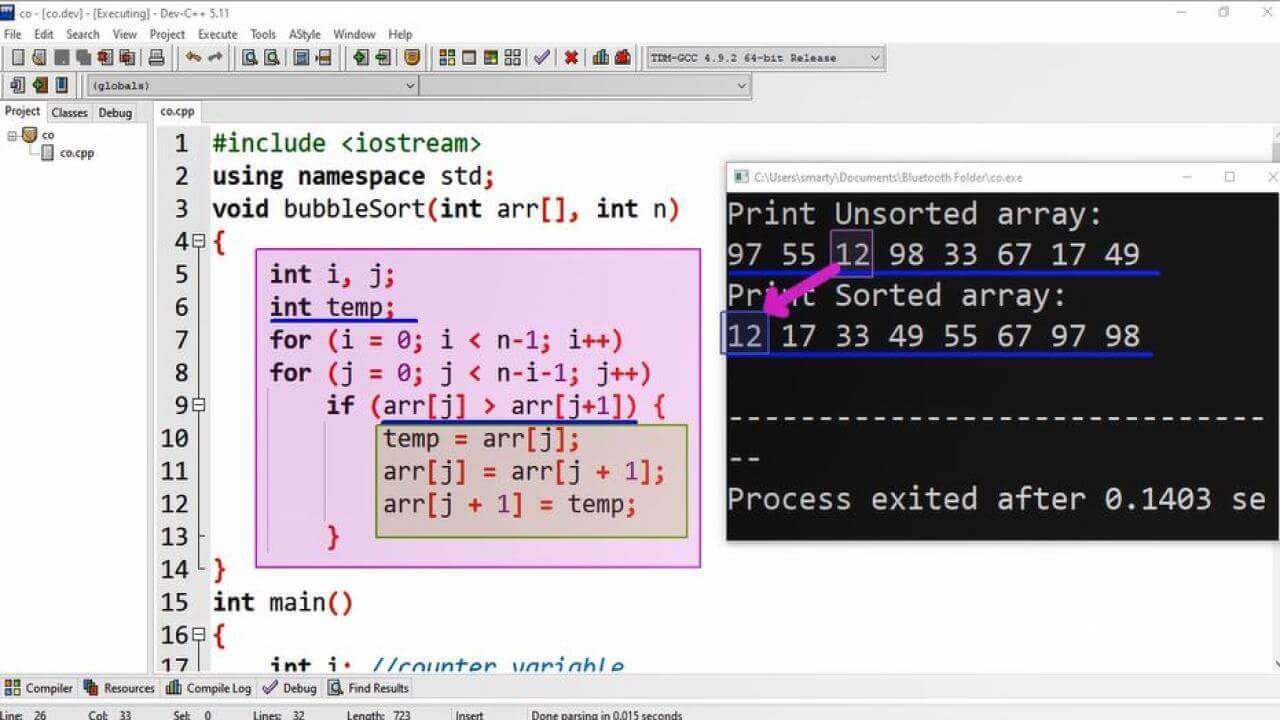# Bubble Sort Program Using Cpp

## Source Code:

#include <iostream>
using namespace std;
void bubbleSort(int arr[], int n)
{
int i, j//Counter variables
int temp// temporary variable for swapping process
for (i = 0; i < n-1; i++)    //outter loop
for (j = 0; j < n-i-1; j++)  // inner loop
if (arr[j] > arr[j+1]) {  // if true then means element need to swap
temp = arr[j];
arr[j] = arr[j + 1];
arr[j + 1] = temp;
// end of if statement
// closing of buuble sort function
int main()
{
int i; //counter variable
int arr[] = {97,55,12,98,33,67,17,49};  // array initialization
int n = sizeof(arr)/sizeof(arr);  // formula to get total elements of array
cout<<"Print Unsorted array: \n";
for (i = 0; i < n; i++) {
cout << arr[i] << " "// printing values of array
}
cout<<endl// print new line in output
bubbleSort(arr, n);  // call of bubble sort function
cout<<"Print Sorted array: \n";
for (i = 0; i < n; i++) {  // printing values of array after sorting
cout << arr[i] << " ";
}
cout << endl;
return 0;
}

## Output:## Working:

In this program example we have defined a function for implementation of bubble sort. This function taking array and size of array as input. In function we declare two variables i and j as counter variables and the third variable temp as temporary variable for swapping vales if these values are not in proper order.

After declaration of variables we use nested loop for traversal of array and swapping elements if these are not in proper order.

In if statement we use > (greater than symbol) for sorting values in Ascending order. Alternatively we will have to use < (less than symbol) for sorting values in Descending order.

In main() function we declare one variable of int data type as counter variable and initialized an array of 8 elements with random values. After this declaration process we print values before sorting and then call bubblesort function and then again print values of array.